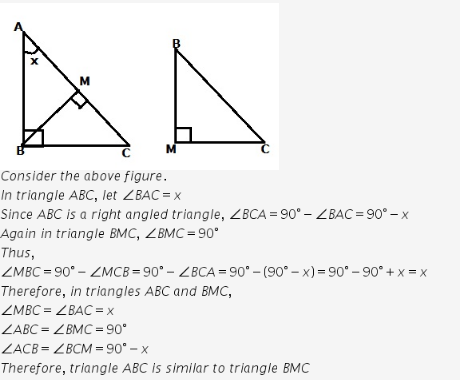# In triangle ABC, if angle B is a right angle and BM is an altitude

In triangle ABC, if angle B is a right angle and BM is an altitude, then triangle ABC is similar to
a) triangle MBC
b) triangle ACB
c) triangle ABC
d) triangle CAB